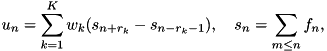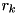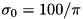A Survey of Gaussian Convolution Algorithms
Stacked integral images Gaussian convolution

A sum of box filter responses, rather than a cascade. More...

## Detailed Description

A sum of box filter responses, rather than a cascade.

This code implements stacked integral images Gaussian convolution approximation introduced by Bhatia, Snyder, and Bilbro and refined by Elboher and Werman. The Gaussian is approximated aswhere the kth term of the sum is effectively a box filter of radius.

The process to use these functions is the following:

1. sii_precomp() to precompute coefficients for the convolution
2. sii_gaussian_conv() or sii_gaussian_conv_image() to perform the convolution itself (may be called multiple times if desired)

The function sii_buffer_size() should be used to determine the minimum required buffer size.

Example
buffer = (num *)malloc(sizeof(num) * sii_buffer_size(c, N));
free(buffer);
References
• A. Bhatia, W.E. Snyder, G. Bilbro, "Stacked Integral Image," IEEE International Conference on Robotics and Automation (ICRA), pp. 1530-1535, 2010. http://dx.doi.org/10.1109/ROBOT.2010.5509400
• E. Elboher, M. Werman, "Efficient and Accurate Gaussian Image Filtering Using Running Sums," Computing Research Repository, vol. abs/1107.4958, 2011. http://arxiv.org/abs/1107.4958

## Data Structures

struct  sii_coeffs_
Parameters for stacked integral images Gaussian approximation. More...

## Macros

#define SII_MIN_K   3
Minimum SII filter order.

#define SII_MAX_K   5
Maximum SII filter order.

#define SII_VALID_K(K)   (SII_MIN_K <= (K) && (K) <= SII_MAX_K)
Test whether a given K value is a valid SII filter order.

## Typedefs

typedef struct sii_coeffs_ sii_coeffs
Parameters for stacked integral images Gaussian approximation.

## Functions

void sii_precomp (sii_coeffs *c, double sigma, int K)
Precompute filter coefficients for SII Gaussian convolution. More...

long sii_buffer_size (sii_coeffs c, long N)
Determines the buffer size needed for SII Gaussian convolution. More...

void sii_gaussian_conv (sii_coeffs c, num *dest, num *buffer, const num *src, long N, long stride)
Gaussian convolution SII approximation. More...

void sii_gaussian_conv_image (sii_coeffs c, num *dest, num *buffer, const num *src, int width, int height, int num_channels)
2D Gaussian convolution SII approximation More...

## Function Documentation

 long sii_buffer_size ( sii_coeffs c, long N )

Determines the buffer size needed for SII Gaussian convolution.

Parameters
 c sii_coeffs created by sii_precomp() N number of samples
Returns
required buffer size in units of num samples

This routine determines the minimum size of the buffer needed for use in sii_gaussian_conv() or sii_gaussian_conv_image(). This size is the length of the signal (or in 2D, max(width, height)) plus the twice largest box radius, for padding.

Definition at line 84 of file gaussian_conv_sii.c.

 void sii_gaussian_conv ( sii_coeffs c, num * dest, num * buffer, const num * src, long N, long stride )

Gaussian convolution SII approximation.

Parameters
 c sii_coeffs created by sii_precomp() dest output convolved data buffer array with space for sii_buffer_size() samples src input, modified in-place if src = dest N number of samples stride stride between successive samples

This routine performs stacked integral images approximation of Gaussian convolution with half-sample symmetric boundary handling. The buffer array is used to store the cumulative sum of the input, and must have space for at least sii_buffer_size(c,N) samples.

The convolution can be performed in-place by setting src = dest (the source array is overwritten with the result). However, the buffer array must be distinct from src and dest.

Definition at line 108 of file gaussian_conv_sii.c.

 void sii_gaussian_conv_image ( sii_coeffs c, num * dest, num * buffer, const num * src, int width, int height, int num_channels )

2D Gaussian convolution SII approximation

Parameters
 c sii_coeffs created by sii_precomp() dest output convolved data buffer array with space for sii_buffer_size() samples src image to be convolved, overwritten if src = dest width image width height image height num_channels number of image channels

Similar to sii_gaussian_conv(), this routine approximates 2D Gaussian convolution with stacked integral images. The buffer array must have space for at least sii_buffer_size(c,max(width,height)) samples.

The convolution can be performed in-place by setting src = dest (the source array is overwritten with the result). However, the buffer array must be distinct from src and dest.

Definition at line 162 of file gaussian_conv_sii.c.

 void sii_precomp ( sii_coeffs * c, double sigma, int K )

Precompute filter coefficients for SII Gaussian convolution.

Parameters
 c sii_coeffs pointer to hold precomputed coefficients sigma Gaussian standard deviation K number of boxes = 3, 4, or 5
Returns
1 on success, 0 on failure

This routine reads Elboher and Werman's optimal SII radii and weights for reference standard deviationfrom a table and scales them to the specified value of sigma.

Definition at line 41 of file gaussian_conv_sii.c.